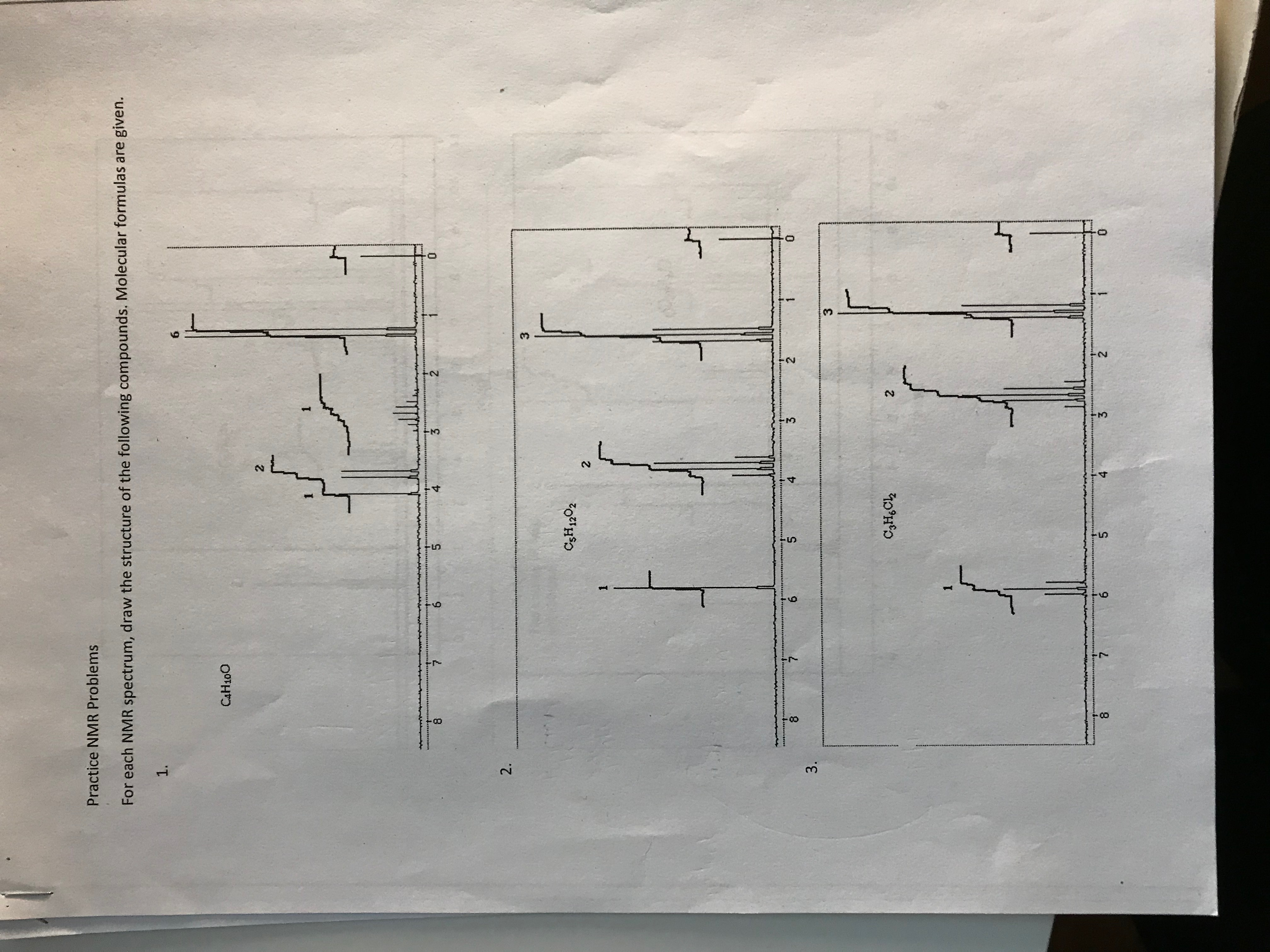# 3.6.2.5.4.2.1.40°H°03.6.3.2.1.CAH1001.6.For each NMR spectrum, draw the structure of the following compounds. Molecular formulas are given.Practice NMR Problems

Question
78 views

The first question is the one I need answered.help_outlineImage Transcriptionclose3. 6. 2. 5. 4. 2. 1. 40°H°0 3. 6. 3. 2. 1. CAH100 1. 6. For each NMR spectrum, draw the structure of the following compounds. Molecular formulas are given. Practice NMR Problems fullscreen
check_circle

Step 1

In H-NMR, the intensity of the peak indicated the number of same types of Hydrogen atoms.

Also, splitting of a peak in H-NMR occurs due to presence of adjacent hydrogen atoms. The splitting of a proton gives (n+1) peaks, where n is the number of adjacent hydrogen atoms.

Step 2

From H-NMR spectrum, we can observe the following:

• A singlet (1 peak) for 1 H at 4.2 which indicates the presence of -OH group. The hydrogen is marked as ‘a’ in the figure.
• A doublet (1+1) for 2 H at around 3.7 indicates the presence of hydrogen (marked as ‘b’ in the figure) that are adjacent to a -CH- group. And this doublet is formed at higher chemical shift value due to the pr...

### Want to see the full answer?

See Solution

#### Want to see this answer and more?

Solutions are written by subject experts who are available 24/7. Questions are typically answered within 1 hour.*

See Solution
*Response times may vary by subject and question.
Tagged in

### Other